Critical Graphene Spin Susceptibility

R. B. Laughlin May 19, 2008

(Submitted as coursework for Physics 373, Stanford University, Spring 2008)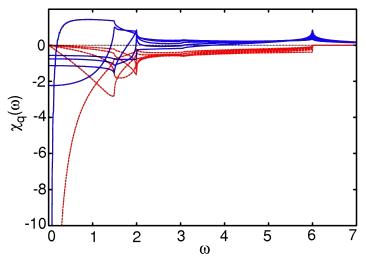Fig. 1: Real (blue) and imaginary (red) parts of renormalized susceptibility at the ordering wavevector (q = 4π/3 along bond) for 5 values of U from zero to Uc. The computer code that generated this graph is available here. I plotted using gnuplot. The plot file is shown here.

This report shows how the calculation for graphene's susceptibity discussed in my previous report can be outfitted with a vertex correction to become a critical susceptibility. In the presence of a Hubbard U, the ladder sum is implemented exactly by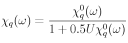where χq0(ω) is the noninteracting susceptibility described in my previous report. The critical value of U is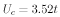For values of U greater than this, the Hartree-Fock equations become unstable to spin polarization, and the ladder sum becomes unphysical when performed with an unpolarized vacuum. However, for all values of U less than this, the unpolarized state is fine. The approach to criticality is signaled by an ω = 0 divergence at the ordering wavector, which in the case of graphene is a near-neighbor reciprocal lattice vector, i.e. a momentum of q = 4π/3 in the direction of one of the bonds. The susceptibility becomes divergent at all three of these simultaneously.

Fig. 1 shows the susceptibility at one of these special wavevectors for 5 values of U increasing from 0 to Uc. One sees a huge transfer of spectral weight to low frequencies, with the real part of χ diverging as 1/ω at criticality.

In Fig. 2 I show the same calculation at nearby wavevectors 3.5&pi/3 and 3π/3. While the real part of χ at ω = 0 is powerfully enhanced at criticality, it is not divergent. However, a much more interesting feature is the sharp pole bound down out of the continuum, with more binding at larger U. This is the precursor of the spin wave of the ordered state. In effect, the spin wave begins to take form before the ordering ever happens! The reason is that, at short length scales, the system can't tell that it isn't ordered. Thus the system has become a "spin liquid," a state that has short-range behavior like that of the ordered antiferromagnet but long-range behavior identical to that of the original noninteracting graphene.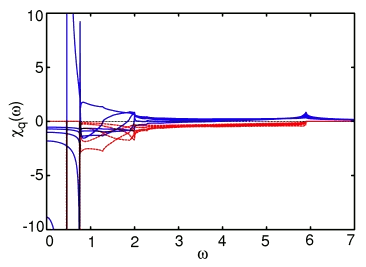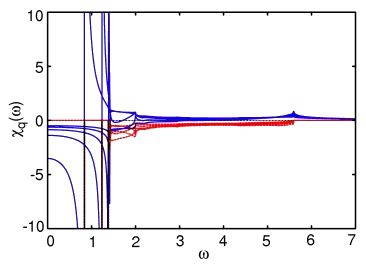Fig. 2: Real (blue) and imaginary (red) parts of renormalized susceptibility at nearby wavevectors (q = 3.5π/3 and 3π/3 along bond) for same 5 values of U from zero to Uc. The computer code that that generated this graph is available here. I plotted using gnuplot. The plot file is shown here.

The LaTeX source of the above equations is available here.